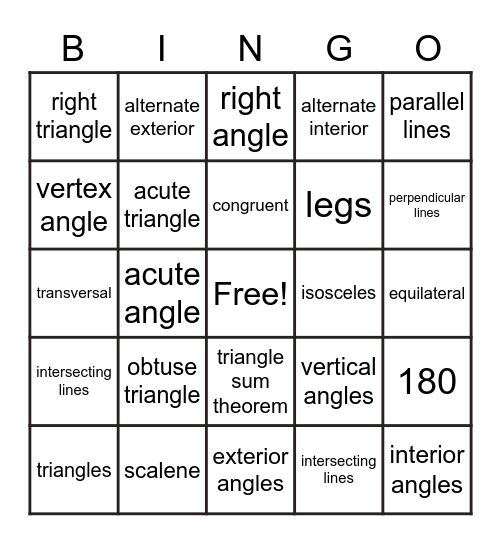# Triangles, Parallel Lines, & TransversalsThis bingo card has a free space and 29 words: triangles, corresponding, acute angle, obtuse angle, right triangle, right angle, acute triangle, obtuse triangle, isosceles, scalene, equilateral, interior angles, exterior angles, intersecting lines, perpendicular lines, exterior angles theorem, triangle sum theorem, intersecting lines, parallel lines, transversal, vertical angles, hypotenuse, legs, vertex angle, alternate interior, alternate exterior, 180, congruent and supplementary.

⚠ This card has duplicate items: intersecting lines (2)

## Play Online